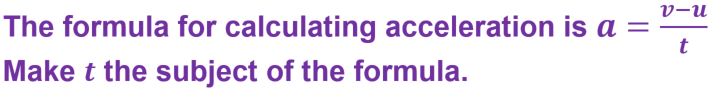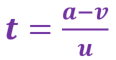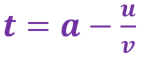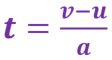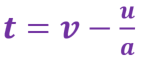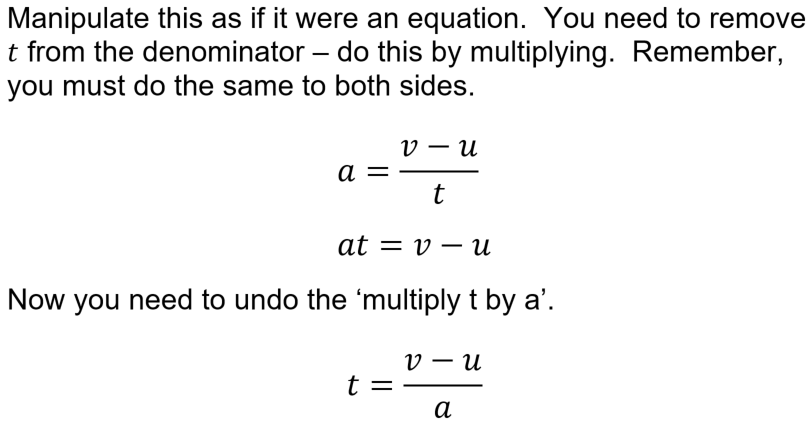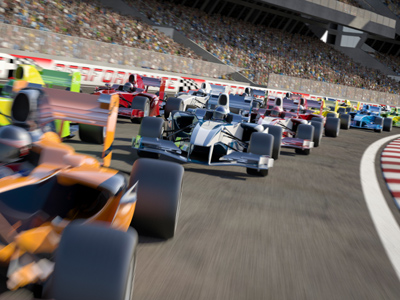Formula One teams need to know all about formulas!

# Formulas and Equations (F)

A formula is a special type of equation that shows how two or more variables (letters) are related. You will already be familiar with some, like the formula for the area of a rectangle. If you know the length and width, you can use the formula to calculate the area. A useful thing about formulas is that you can rearrange them to work out the value of another variable. So if you know the area of a rectangle and its width, you can calculate the length. Test your skills in using and rearranging formulae in this GCSE Maths quiz.

You have to know how to SUBSTITUTE a value into a formula – you do this when you are told what value a variable has. Every time the letter appears in the formula, replace it with the number, remembering to keep the same relationships such as multiplying or adding.

The ‘subject’ of a formula is the variable that everything else is equal to. It is usually on the left-hand side of the equal sign, but it doesn’t have to be. You may be asked to ‘change the subject of the formula’ – this means you have to carry out algebraic manipulation to rearrange the variables. This is similar to solving equations, in that you are trying to remove everything from the variable that is to be the subject. However, they don’t just disappear, but have to appear on the other side of the equal sign, retaining the correct relationships with the other variables.

Question 1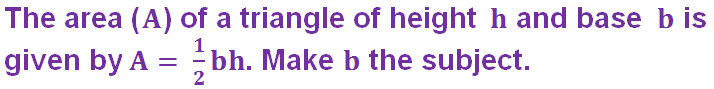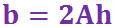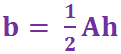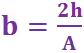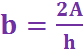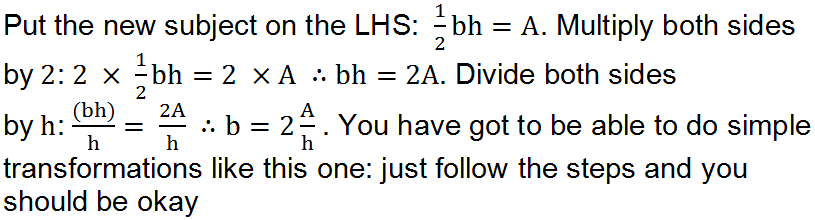Question 2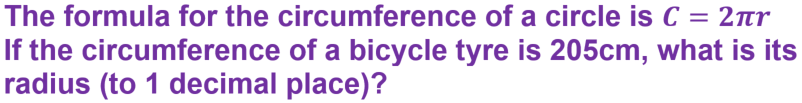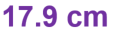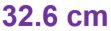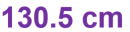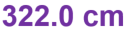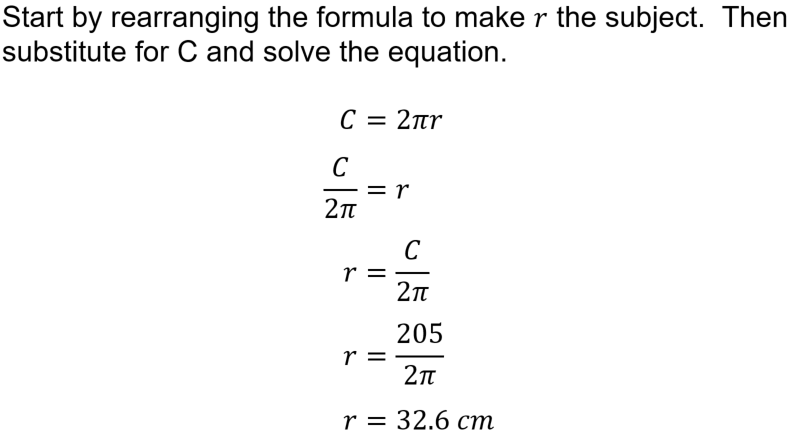Question 3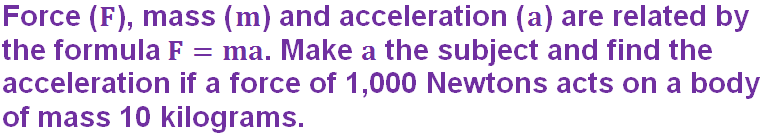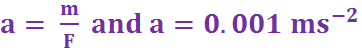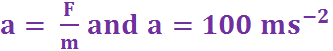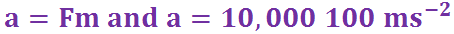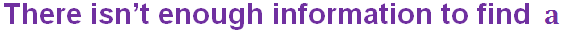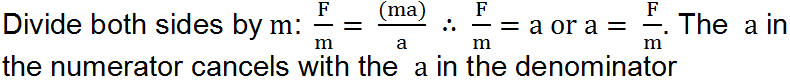Question 4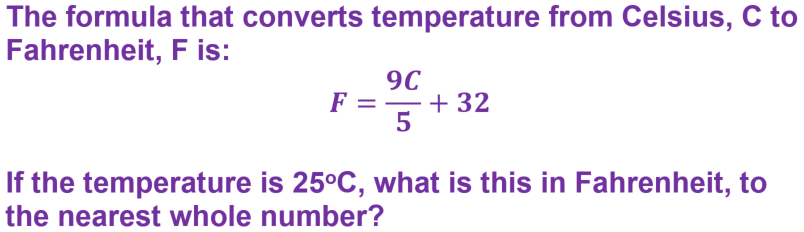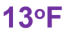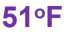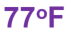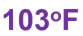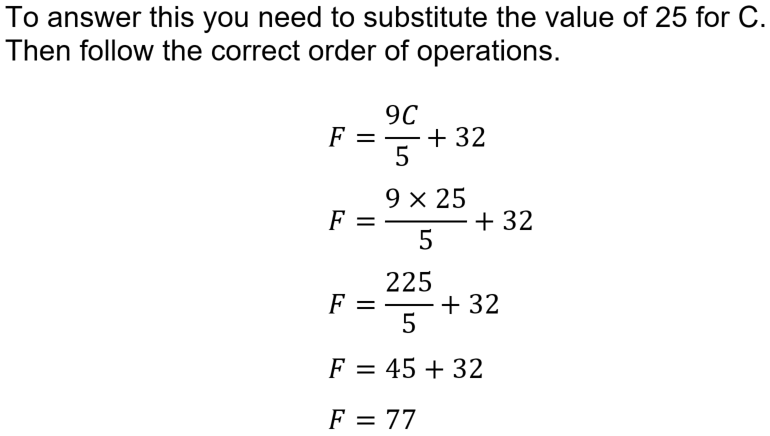Question 5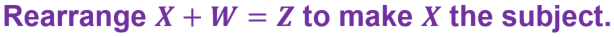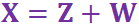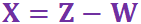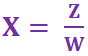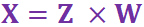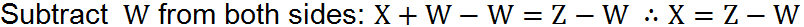Question 6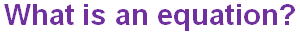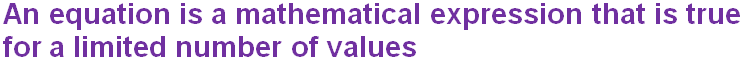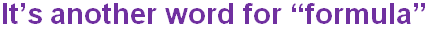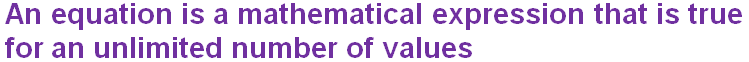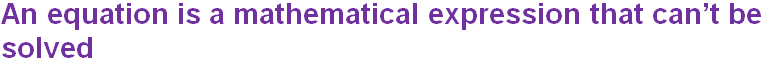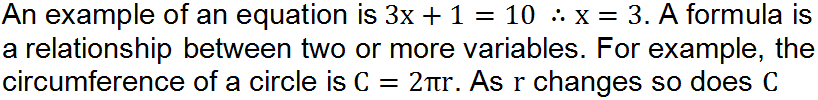Question 7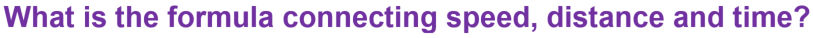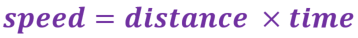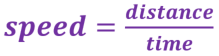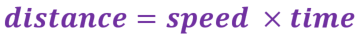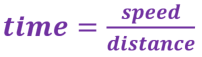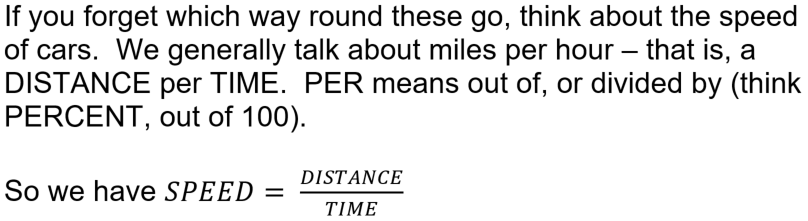Question 8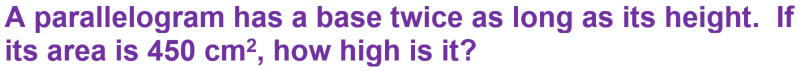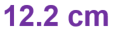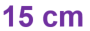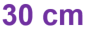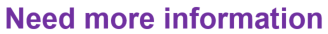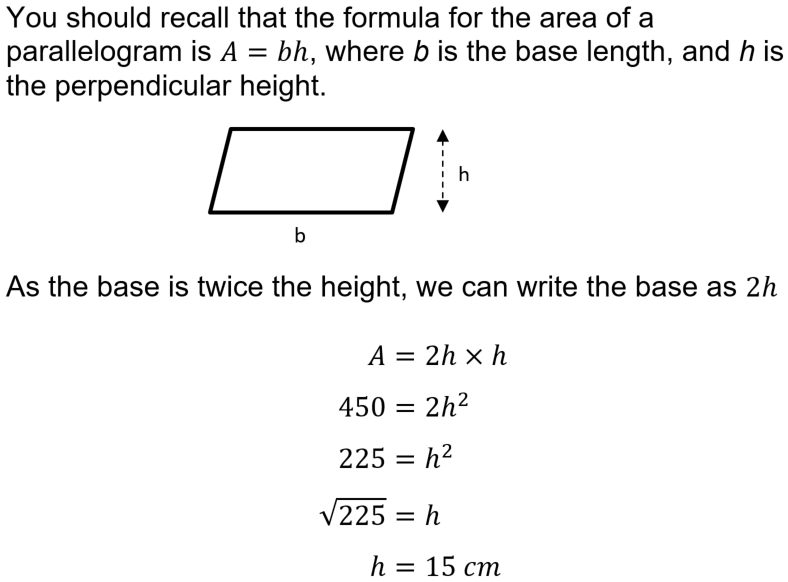Question 9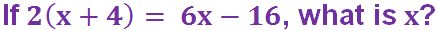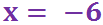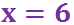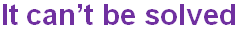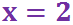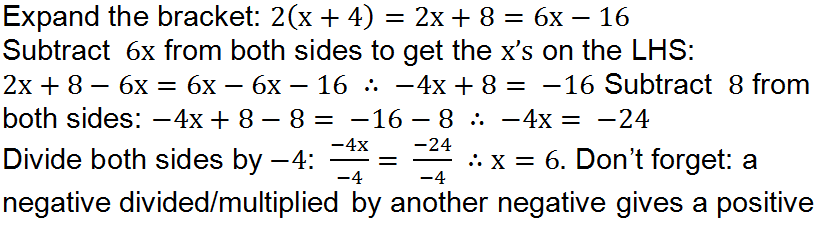Question 10